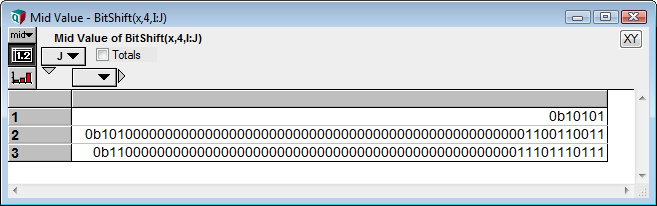# BitShift

(diff) ← Older revision | Latest revision (diff) | Newer revision → (diff)

## BitShift(x, shift, left, I, rotate, bit )

Shifts the bits in an integer value «x» bit «shift» positions to the right, or by «shift» positions to the left when «left» is specified as true. Alternatively, «shift» can be negative to invert the shift direction.

When an array is provided and the index «I» is specified, the bits are shifted to the adjacent cell. Each integer in each cell is considered to have 64 bits.

When «rotate» is true, the bits are rotated, so that a bit that shifts off the right edge is used for the value of the bit shifted in on the left.

When «rotate» is not specified, you can specify the value for new bits in the «bit» parameter, which must be either 0 or 1.

When neither «rotate» nor «bit» are specified, then 0 bits are shifted into the result when shifting left, but when shifting right, the most significant bit (the 64th bit) is used, which has the effect of preserving the sign of the integer. This default is equivalent to integer division by powers of 2 when shifting right, and multiplication by powers of 2 when shifting left.

## Examples

To make the effect of the function obvious, integers are written here in binary notation.

BitShift(0b110101011100, 5) →
0b1101010
BitShift(3420, 5) →
106
BitShift(0b110101011100, 5, left: true) →
0b110101011100000
BitShift(3420, 5, left: true) →
109440

Negative numbers have leading 1 bits, and are 64 bits in length. Shifting right shifts 1s into the left side.

BitShift(-170, 5) →
0b1111111111111111111111111111111111111111111111111111111111111010 = -6

Or you can shift in zero bits:

BitShift(-170, 5) →
0b0000011111111111111111111111111111111111111111111111111111111010 = 576460752303423482
BitShift(0b1101010111, 3, rotate: true) →
0b1110000000000000000000000000000000000000000000000000000001101010

Next, shifting across cells of an array:

x :=BitShift(x, 4, I: J) →BitShift(x, 4, I: J, rotate: true) →### Convert Hexadecimal to RGB numeric values

Colors are commonly defined with three channels for information: Red, Green, and Blue. The BitShift function offers a straightforward way to convert colors defined by a hexadecimal string to numerical RGB triplet.

Local hex_val := 0x0079BF
Local R := BitShift( hex_val, 16 ) → 00
Local G := BitShift( hex_val, 8 ) - BitShift( R, 8, left: True ) → 79
Local B := hex_val - BitShift( R, 16, True ) - BitShift( G, 8, True ) → BF

Displayed as integers, these values are [R, G, B] = [0, 121, 191]

## History

Introduced in Analytica 5.0.